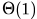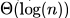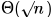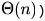Courses

# Consider a carry look ahead adder for adding two n-bit integers, built using gates of fan-in at most two. The time to perform addition using this adder isa)b)c)d)Correct answer is option 'B'. Can you explain this answer? Related Test: Boolean Operations MCQ Quiz - 1

## Computer Science Engineering (CSE) Question

Rachika Grewal answered Jul 05, 2018
Look ahead carry generator gives output in constant time if fan-in = number of inputs.

For Example:

It will take O(1) to calculate
c4 = g3 + p3g2 + p3p2g1 + p3p2p1g0 + p3p2p1p0c0c4
= g3 + p3g2 + p3p2g1 + p3p2p1g0 + p3p2p1p0c0,
if OR gate with 5 inputs is present.
And, if fan-in != number of inputs then we will have delay in each level, as given below.

If we have 8 inputs, and OR gate with 2 inputs, to build an OR gate with 8 inputs, we will need 4 gates in level-1, 2 in level-2 and 1 in level-3. Hence 3 gate delays, for each level.

Similarly an n-input gate constructed with 2-input gates, total delay will be O(log n).